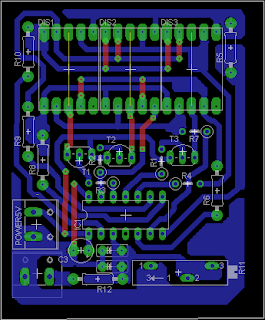Browse over 10,000 Electronics Projects

# 30 volts Panel Volt Meter Using PIC MCUBROWSE

This is a simple application of internal 10-bit ADC(analog to digital  converter) of PIC16F676 microcontroller.you can use this  circuit  to measure  up to 30 v dc. the possible  applications are on bench top power supply or as a panel meter in various system.
Circuit description
MICROCHIP’S PIC16F676 is the heart and brain of this circuit .the internal adc of the mcu with a resistor network voltage  divider is used to measure the input voltage . then 3 digest of comm anode 7 segment display is used to display final converted voltage. as you can see in the schematic the displays are multiplexed with each other . means we switch on one display and put the corresponding digit on this while other two displays are off this cycle go for each of the display.
you can find more about driving multiplexed 7 segment led display from a pic mcu in application note from microchip AN557 Four Channel Digital Voltmeter with Display and Keyboard
in my circuit the refresh rate is about 50hz.

Voltage Divider Front End

as you can seen in the schematic the 47k resistor and 10 k trim pot is connected ias a voltage divider configuration  .we all know very well that by default pic micro controller ADC reference voltage is set to vcc(+5v in this case) . so what we have to do is make such voltage divider that can divide out maximum range 30 volts to 5 volts . so we need is Vin/6 ==> 30/6 =5v   voltage divider . and to keep as less as possible attenuation on the under test voltage we have to keep the voltage divider resistor value in few thousand ohms because it takes very little current from the target but as much to drive adc of pic.

calculation
10bit adc resolution we get 1023 maximum count
with 5 v reference  we get  5/1023 = 0.0048878 V/Count
means if the adc count is 188 then input voltage is 188 * 0.0048878 == 0.918 volts

but now with the voltage divider  the maximum  voltage  is 30v so the calculations
will be  30/1023= 0.02932 volts/count
if now we get 188 then 188*0.02932==5.5 Volts

you can also increase  or decrease  the range by changing resistor network and the calculations a little bit.

the capacitor 0.1uf makes the adc input a bit stable because 10bit adc is really sensitive .
the 5.1v zener will provide over votage protection to the internal adc because it wont allow voltage more than 5.1v.
Accuracy and calibration
overall  accuracy of this circuit is great but it totally depends on the values of 47K resistor and 10k trim pot . as fine as you can go by adjustment of the trim pot your accuracy goes fine.

Advertisement

calibration of this circuit is done by adjustment of the 10k trimpot around value of 7.5k or so .
all you have to do is take any standard power like 5v or 12v and apply that to the input of the resistor network and adjust the trimpot until you get correct value on the display

PCBSoftware  Sorce code and Firmware
the sotware is written in c and complied using hi-tech compiler

click here download firmware  and source code

BROWSE

Top